I recently came across an interesting use case for the Groupby function in DAX, and while doing so, thought it would also make a great example for explaining evaluation context in DAX.

Consider the following table of data showing purchase requirements for two products, from multiple suppliers.

Each product/SKU combination can only be bought in a certain minimum order quantity, so even if you need to purchase only 3 units of product A in an XS stock keeping unit (SKU), you need to buy at least 100 to comply with the rule.

 Date Supplier Product SKU Min unit qty Qty Required 31/05/2018 Supplier2 A XXS 100 14 31/05/2018 Supplier2 A XXS 100 20 31/05/2018 Supplier1 A XS 100 14 30/06/2018 Supplier1 A XS 100 3 30/06/2018 Supplier2 A S 100 64 30/06/2018 Supplier2 A M 80 4 30/06/2018 Supplier1 B XXS 100 91 30/06/2018 Supplier1 B M 80 9 30/06/2018 Supplier1 B L 80 23 30/06/2018 Supplier2 B L 80 18 30/06/2018 Supplier1 B XL 60 18 30/06/2018 Supplier1 B XL 60 18 30/06/2018 Supplier1 B XXL 60 7

With a bit of manipulation, you could present the data in the following pivot table, using a measure (Base qty) to aggregate the quantity required with a simple sum: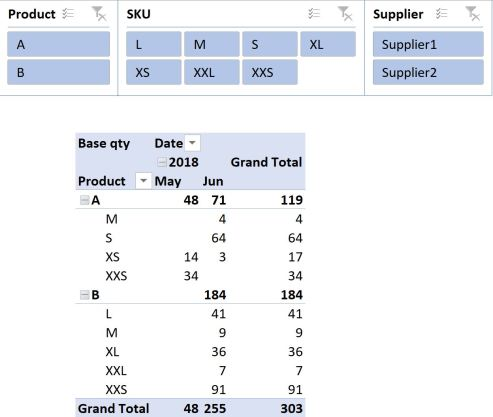Next, you might want to work out an order plan that complies with your minimum order requirements. In its basic form (I’ll use the DAX Variable syntax), this equation would look something like this, where [Minimum Unit Qty] = MIN(Table1[Min unit qty]) ♦:

```Qty in unit packs 1 =
VAR frac =
DIVIDE ( [Base qty], [Minimum Unit Qty] )
VAR Unitqty =
ROUNDUP ( frac, 0 )
RETURN
Unitqty * [Minimum Unit Qty]
```

♦ You could have used the MAX or the SUM function here too.

Adding this measure to the pivot table and removing date information so we just look at the total amount for all time, yields the following result: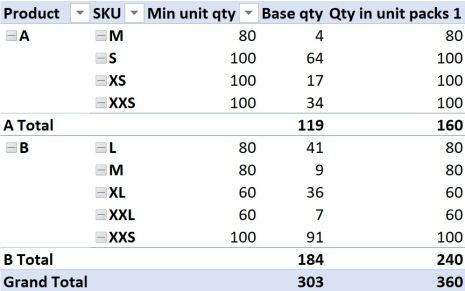If it isn’t immediately obvious, the totals of our new measure don’t add up to what we might have hoped for. This is because our new measure is evaluated in FILTER context.

What does that mean?

While there is no single minimum unit quantity for product A across all SKU’s, (there are multiple), our measure for aggregating the minimum order quantity tells it to look for the MINIMUM value across all Product A’s in the current filter context. In the example above, for Product A, that is 80 units when we consider the total quantity for Product A.

While we know of course that this is not at all relevant to the total quantity across all Product A’s, it does explain the result the formula is giving us. 119 units divided by 80, rounded up to zero and multiplied by 80 gives 160, so that is how the totals are calculated. In the same way, you can confirm how the numbers 240 and even the 360 were arrived at. That is filter context in action.

Coming back to my previous comment where I said you could have also used the MAX function to aggregate the minimum order quantity, the result would just have used the value 100 instead of 80 or 60, in the cases of Product A or B, but your totals would have been calculated in a similar manner.

The SUM function would operate in the same way, except it would use the sum of the minimum order quantities as the denominator and give us a total of 360, which is what we want, but this is purely a coincidence, because the result would have still been calculated using filter context, and if the data were a little different, or even if we just had more rows of data, you would quickly find that the totals don’t “add up”.

Okay, so we know we don’t want filter context when looking at totals, but how can we change FILTER context into ROW context?

The first thing that popped into my head was SUMX, because I know that this function forces filter context into row context, and I often use it to make the totals in pivot tables “add up” to what I want to see, and to what makes sense to me.

Let’s modify our equation to use SUMX then (we’ll ditch the Variable syntax for now because we have to refer to “naked columns” for this SUMX to work):

```Qty in unit packs 2 =
SUMX (
Table1,
ROUNDUP ( Table1[Qty Required] / Table1[Min unit qty], 0 )
* Table1[Min unit qty]
)
```

Adding this measure to our pivot table yields some more confusing results: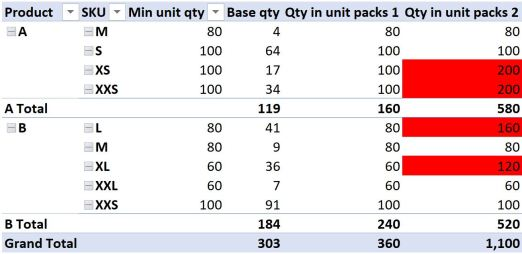The results in the first couple of lines look fine, however the third and fourth lines might give you reason to frown. We only need 17 units (line 3), and the minimum order quantity is 100, yet the formula returns a value of 200? How can that be? All we did was apply a SUMX to the value our measure returns. At least the totals appear to be adding things up as we expected.

The reason for the measure returning 200 in the third line instead of 100 has to do with the fact that the 17 units in our underlying data is made up from two different dates; 14 units in May and 3 units in June (see the very first pivot table). These dates are not shown in our current pivot table, but they still exist in the underlying data source. Our new SUMX formula honours this “hidden” row context, as can be revealed by adding the Date field to the pivot table filtered to show product A and the XS SKU: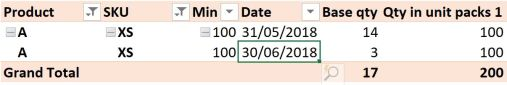The formula therefore does the calculation on both instances, or both ROWS in our underlying data.

What about the fourth line then? The quantity is only valid for one date, and comes from the same supplier too, so why does this result give 200?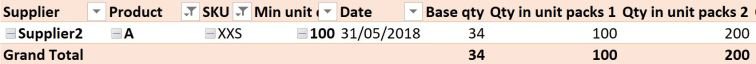To get the answer in THIS case, we have to dig a little deeper. Looking at the source data table, we can see that the 34 units in May are comprised of two different lines, or rows, for 14 and 20 units respectively. Once again, SUMX has performed our calculation on both rows of this data individually before adding the result together, because we have forced context transition to ROW context. The results for Product B can be explained in a similar way, noting that for the SKU = “L”, the product is sourced from two different suppliers, so technically 160 is correct and 80 is wrong! Product B SKU “XL” is made up of two duplicate rows again, like Product A SKU “XXS”.

The GOOD news is that we have confirmed that yes, SUMX changes FILTER context into ROW context, because it now evaluates the formula for each and every row in our source table, and the totals at least “add up” as we would expect. The BAD news is that SUMX is now giving us “wrong” values for some lines.

Okay, you might say, well why don’t you just go back to Power Query or SQL and group the data to get rid of duplicate rows and the date information, and use this as your new source data?

This would work, but we would then lose the ability to apply a date filter to our data after we load it to the data model.

What if we could group the data “on the fly”, doing the grouping only on the selected subset of data as filtered by slicers and other filters?

Enter GROUPBY

The GROUPBY function in DAX can be used to calculate a new table “on the fly”, where we group our underlying data source to only include the columns we specify, while honouring existing external filters.

We can then use SUMX, AVERAGEX, MAXX or any other such iterator to aggregate the numbers in the current group (table) that we are calculating on the fly. To refer to this virtual table, the syntax CURRENTGROUP() is used.

So what does that look like in our example? I want to group this data to exclude date information. We’ll use the DAX Variable syntax again:

```Qty in unit packs 3 =
VAR Groupedtable =
GROUPBY (
Table1,
Table1[Supplier],
Table1[Product],
Table1[SKU],
Table1[Min unit qty],
"Groupedvalue", SUMX ( CURRENTGROUP (), Table1[Qty Required] )
)
VAR something =
SUMX (
Groupedtable,
ROUNDUP ( DIVIDE ( [Groupedvalue], Table1[Min unit qty] ), 0 )
* Table1[Min unit qty]
)
RETURN
something
```

♣ You don’t have to group data using columns from the same table; you can use columns in lookup tables, but the first table in the GROUPBY function would be the table containing the numeric data you want to group.

While verbose, the result gives us exactly what we need: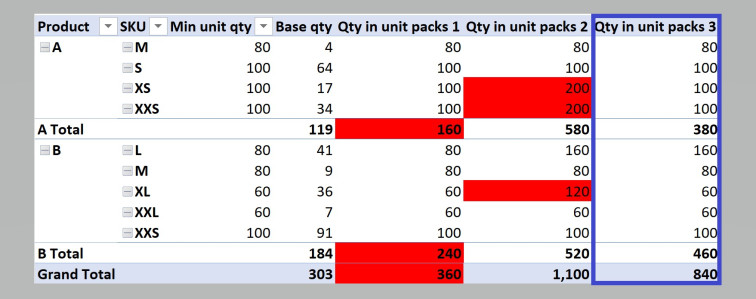Could I have used the SUMMARIZE function?

Absolutely, but this is not a post about SUMMARIZE. We can use a very similar syntax, with the exception of the CURRENTGROUP() reference:

```Qty in unit packs 4 =
VAR Summarizedtable =
SUMMARIZE (
Table1,
Table1[Supplier],
Table1[Product],
Table1[SKU],
Table1[Min unit qty],
"Summarizedvalue", SUMX ( Table1, Table1[Qty Required] )
)
VAR something =
SUMX (
Summarizedtable,
ROUNDUP ( DIVIDE ( [Summarizedvalue], Table1[Min unit qty] ), 0 )
* Table1[Min unit qty]
)
RETURN
something
```

So how are they different?

Summarize does an implicit CALCULATE to each extension column it adds, whereas GROUPBY does not. GROUPBY is also tipped to be very performant, and personally I found the syntax more palatable than traditional explanations of SUMMARIZE and its variations. But neither of those considerations are important for our current dataset.

The really great thing about using GROUPBY or SUMMARIZE to recalculate the grouped table “on the fly”, is that you can still apply an external filter to the data (such as a date filter, or a transaction ID filter, if we had that detail in our data set) even if it isn’t included in the calculated group, and it will respond appropriately. That is really what enthused me to write this post.

I’m sure there are other implications to consider for choosing between GROUPBY and SUMMARIZE – let me know in the comments – but hopefully you’ve learned something.

# Power to the Masses

Copyright 2019 Power to the Masses.